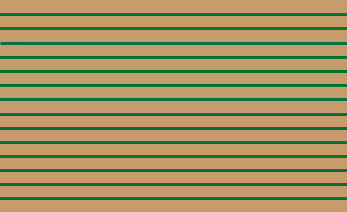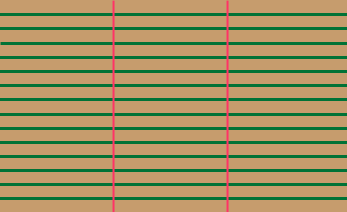I am having a complete brain freeze! Help! HOW DO I CONVERT A FRACTION TO A DECIMAL? (E.G,. 8/14 to ? ) And HOW DO I DIVIDE A FRACTION (e.g., 1/14 divided by 3) and then convert that answer to a decimal? I have several conversion tables they cover the “normal” fractions, etc. ½, 1/3, 5/8 etc. Diane Diane, You convert a common fraction to a decimal by division. For example for 8/14 divide 8 by 14 to get 0.5714... I have a garden with 14 rows of carrots.I plan to dig one row tonight so that will give me one fourteenth of the carrots. I will then take the carrots I have dug and divide by 3 in order to share them with my son and the food bank. What fraction of the entire crop of carrots am I going to give to the food bank? In other words what is 1/14 divided by 3? Divide the entire garden by 3 to get 143 = 42 pieces (each one third of a row in length).The food bank gets one of these pieces, that is 1/42 of the carrot crop. Thus 1/14 divided by 3 is one third of 1/14, which is 1/31/14 = 1/(314) = 1/42 To convert to a fraction divide 1 by 42 so 1/42 = 0.0238... I hope this helps. Penny